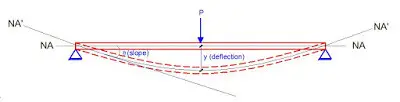Two Approaches to the Calculation of Deflection in Eurocode 2The verification of deflection is an important serviceability check in reinforced concrete design. Most designs codes adopt the ‘deemed to satisfy’ rules which limit the deflection to an acceptable depth using Basic Span/Effective depth ratio. This is generally more frequently employed than carrying out the rigorous exact calculation of deflection which is however not
very reliable.

Eurocode 2 has its own set of deemed to satisfy rules, and there are two approaches to calculation of deflection which yields the same result. It is not like that they are two different formulars, but they are just one formular expressed in two different ways. This post shows the two approaches, so that you can select the one you deem fast enough for carrying out your manual calculations.

According to Clause 7.4.2 of EN 1992-1-1, we can verify deflections in the absence of exact calculations using deemed to satisfy basic span/effective depth (limiting deflection to depth/250);

On the Deformation of Statically Indeterminate Frames Using Force Method

Lateral Deflection of Multi-Storey Buildings Under the Action of Wind Load

(a) BY USING THE FIRST APPROACH
Actual L/d must be ≤ Limiting L/d × βs

The limiting basic span/ effective depth ratio is given by;
L/d = K [11 + 1.5√(fck) ρ0/ρ + 3.2√(fck ) [(ρ0 / ρ) – 1](3⁄2) ] if ρ ≤ ρ0 ———– (1)

L/d = K [11 + 1.5√(fck) ρ0/(ρ – ρ’) + 1/12 √(fck) (ρ0/ρ)(1⁄2) ] if ρ > ρ0 ———— (2)
Where;
L/d is the limiting span/depth ratio
K = Factor to take into account different structural systems
ρ0 = reference reinforcement ratio = 10-3√(fck)
ρ = Tension reinforcement ratio to resist moment due to design load
ρ’ = Compression reinforcement ratio

The value of K depends on the structural configuration of the member, and relates the basic span/depth ratio of reinforced concrete members. This is given in the Table below;

βs = (500 Asprov)/(Fyk Asreq) ———- (3)

(b) BY USING THE SECOND APPROACH
L/d ≤ Basic ratio × αs × βs
Where the basic ratio = 20K

Modification factor αs which depends on the concrete strength fck and reinforcement percentages, is given by:

For 100As/bd < 0.1fck0.5;
αs = 0.55 + 0.0075fck/(100As/bd) + 0.005fck0.5[ fck0.5/ (100As/bd) – 10]1.5 —————- (4)

For 100As/bd ≥ 0.1fck0.5;
αs = 0.55 + 0.0075fck/[100(As – As’)/bd) + 0.013fck0.25 (100As’/bd)]0.5 (for doubly-reinforced sections) —————- (6)

αs = 0.55 + 0.0075fck/(100As/bd) (for singly-reinforced sections) —————- (7)

Modification factor βs = 310/σs —————- (8)
Where:
σs is the stress in the tension reinforcement under the characteristic loading. It will normally be conservative to assume;
βs = (500/fyk)(As,prov/As,req) for values of (As,prov/As,req)≤ 1.5 —————- (9)

Important hints on the calculation of deflection using both approaches;

(1) For flanged sections with b/bw ≥ 3, the basic ratios for rectangular sections should be multiplied by 0.8. For values of b/bw < 3, the basic ratios for rectangular sections should be multiplied by (11 – b/bw)/10

(2) The ratio should be based on the shorter span for two-way spanning slabs, and the longer span for flat slabs.

(3) For beams and slabs, other than flat slabs, with spans exceeding 7 m, which support partitions liable to be damaged by excessive deflections, the basic ratio should be multiplied by 7/span. For flat slabs, where one or both spans exceeds 8.5 m, which support partitions liable to be damaged by excessive deflections, the basic ratio should be multiplied by 8.5/span.

SOLVED EXAMPLE

For the example above we check the deflection for span D-E.
fck = 35 N/mm2; fyk = 460 N/mm2; Effective depth = 840mm; Effective width of flange (Beff) = 1650mm; As,req = 1850 mm2; As,prov = 2101 mm2

BY APPROACH 1

ρ = As,prov /Beffd = 2101 / (1650 × 840) = 0.0015158;
ρ0 = reference reinforcement ratio = 10-3√(fck) = 10-3√(35) = 0.005916
Since if ρ ≤ ρ0;

L/d = K [11 + 1.5√(fck) ρ0/ρ + 3.2√(fck) (ρ0 / ρ – 1)(3⁄2)

k = 1.3 (One end continuous)

L/d = 1.3 [11 + 1.5√(35) × (0.005916/0.001516) + 3.2√(35) × [(0.005916 / 0.001516) – 1](3⁄2)
L/d = 1.3[11 + 34.630 + 93.608] = 181.00

βs = (500 Asprov)/(Fyk Asreq) = (500 × 2101) / (460 × 1850 ) = 1.234

Check b/bw = 1650/300 = 5.5
Since b/bw ≥ 3, multiply L/d by 0.8
Since span is greater than 7m, also multiply by 7/span

Therefore limiting L/d = 1.234 × 0.8 × (7/8) × 181.04 = 156.382
Actual L/d = 8000/840 = 9.523

Since Actual L/d < Limiting L/d, deflection is satisfactory.

BY APPROACH 2

100As/bd = (100 × 2101) / (1650 × 840) = 0.1516
Check 0.1fck0.5 = 0.1 × (35)0.5 = 0.5916

Since For 100As/bd < 0.1fck0.5

αs = 0.55 + 0.0075fck/(100As/bd) + 0.005fck0.5[ fck0.5/ (100As/bd) – 10]1.5
αs = 0.55 + [(0.0075 × 35) /(0.1516)] + [0.005 × (35)0.5 × [(350.5/0.1516) – 10]1.5 = 0.55 + 1.7315 + 4.625 = 6.9065

Basic ratio = 20k = 20 × 1.3 = 26

βs = (500/fyk)(As,prov/As,req) = (500/460) × (2101/1850) = 1.234

Check b/bw = 1650/300 = 5.5
Since b/bw ≥ 3, multiply L/d by 0.8
Since span is greater than 7m, also multiply by 7/span

Therefore limiting L/d = 1.234 × 0.8 × (7/8) × 26 × 6.9065 = 155.111
Actual L/d = 8000/840 = 9.523

You can verify that the two methods can be used, with the second method being more approximate.